# AP Calculus BC Review: Ratio and Root Tests

The Ratio and Root Tests are indispensable tools for finding out whether a series converges or diverges. These tests also play a large role in determining the radius and interval of convergence for a series of functions.

In this article, we will discuss how the Ratio and Root Tests work. In addition, you’ll see a few examples from the AP Calculus BC exam.

## The Ratio and Root Tests

Both the ratio and root tests are basically comparing how closely your series might be to a geometric series.

Recall that a geometric series is a series in which the terms have a common ratio.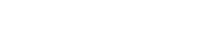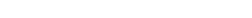The test for convergence for a geometric series is very simple. Let r be the common ratio.

• If |r| < 1, then the geometric series converges.
• If |r| ≥ 1, then the geometric series diverges.The area of fractals like the Koch Snowflake can be found by evaluating a convergent geometric series.

### The Ratio Test

Now suppose that you have a general infinite series like the one below.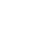A typical series will not have a common ratio. In other words, if you divided an+1 by an, the values you get would depend on n. (In the case of a geometric series, an+1 / an = arn+1 / (arn) = r, which is a constant.)

So, to get around the fact that there is no common ratio, what we do is to take the limit. Then we will use that limiting value to decide whether our series converges or not.

In fact, the Ratio Test determines absolute convergence. (For more details, check out: AP Calculus BC Review: Absolute and Conditional Convergence.)

Ratio Test. Let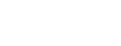.

• If L < 1, then the series converges absolutely.
• If L > 1, then the series diverges.
• However, if L = 1, then the ratio test is inconclusive. (And then other test(s) would be needed to determine whether this series converges or diverges.)

### The Root Test

The Root Test also serves to isolate a potential common ratio, but does so in a different way. This time, we take the nth root of the general term and then allow n to go to infinity.

The inequalities are still the same, though. We still have to test whether the limit is less, greater, or equal to 1.

Root Test. Let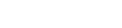.

• If L < 1, then the series converges absolutely.
• If L > 1, then the series diverges.
• However, if L = 1, then the ratio test is inconclusive. (And then other test(s) would be needed to determine whether this series converges or diverges.)

So how is the nth root able to extract information about a potential common ratio? Let’s take a look at how the limit behaves when you actually have a geometric series, Σarn.The limit is L = |r|, which is the common ratio! (Well, technically, it’s the absolute value of the common ratio, but close enough.)

#### Three Useful Limits for Root Test

It can be tricky to deal with a limit of the nth root of some quantity. The following three properties will make your life a lot easier:The Ratio and Root Tests can help to find the radius of convergence for a series of functions. Here’s how it works:

1. Set up the limit L involving the general terms, as outlined above.
2. If L = 0, then the radius of convergence is infinite (R = ∞).
3. If L = ∞, then the radius is zero (R = 0).
4. Otherwise, if L is nonzero and finite, then set up the inequality L < 1, and solve for the expression |xc|. The numerical value that shows up on the right side of the inequality is equal to R.

## Examples

### A Numerical Series

Determine whether the following series converges or diverges.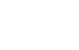#### Your Intuition Might Deceive You

When it comes to sequences and series, often our intuition can be misleading. For example, if you write out the first three terms of this series, you get:

For n = 0, we have 3(0!)/(02 + 1) = 3(1)/1 = 3.

Then for n = 1, we have 3(1!)/(12 + 1) = 3(1)/2 = 1.5.

And for n = 2, the value is 3(2!)/(22 + 1) = 3(2)/5 = 1.2.

It seems that the terms are just deceasing as n increases, right? But the terms actually turn around and start increasing after a while!Surprised cat is surprised.

For n = 3, we have 3(3!)/(32 + 1) = 3(6)/10 = 1.8.

Then for n = 4, the term is 3(4!)/(42 + 1) = 3(24)/17 = 4.235.

In fact, the terms themselves become unbounded as n → ∞. That alone indicates that the series diverges (if you can prove it).

But let’s see how Ratio or Root Test works in this case.

#### Solution

Usually when there are factorials in the general term, the Ratio Test is easier to apply than the Root Test.Now, because the limit is greater than 1 — and really, how much greater than 1 could you get than infinity!? — we conclude that this series diverges.

### When the Tests Fail

Determine whether the alternating harmonic series converges or diverges.#### Solution

You can pick either the Ratio or Root Test. I’ll set up the Ratio Test for you.This time, the limit is exactly 1. That means that the Ratio Test is inconclusive. It won’t help to try the Root Test instead — you’ll get the same result.

So what do we do now?

You’ll have to pick a different convergence test. I’d recommend the Alternating Series Test (AST), because this series is alternating, after all.

Examining the absolute value of the general term, 1/n, we see that it does decrease down to zero (as n → ∞). Therefore, by the AST, the alternating harmonic series converges.

### Finding the Radius of Convergence

Find the radius of convergence for the following series.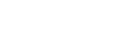#### Solution

This one is just begging for Root Test!Since the limit was neither 0 nor infinity, we have to set the expression less than 1 and solve.The last line gives two pieces of information. Both are derived from the general template, |xc| < R, where c is the center, and R is the radius of convergence.

• The radius of convergence is R = 1/3.
• The center of the power series is c = -2/3.

#### Improve your SAT or ACT score, guaranteed. Start your 1 Week Free Trial of Magoosh SAT Prep or your 1 Week Free Trial of Magoosh ACT Prep today!## Author

•Shaun earned his Ph. D. in mathematics from The Ohio State University in 2008 (Go Bucks!!). He received his BA in Mathematics with a minor in computer science from Oberlin College in 2002. In addition, Shaun earned a B. Mus. from the Oberlin Conservatory in the same year, with a major in music composition. Shaun still loves music -- almost as much as math! -- and he (thinks he) can play piano, guitar, and bass. Shaun has taught and tutored students in mathematics for about a decade, and hopes his experience can help you to succeed!